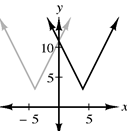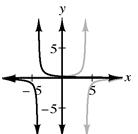### Home > CCA2 > Chapter 2 > Lesson 2.2.3 > Problem2-126

2-126.

For each of the following functions sketch the graph of the original and of $y=f(-x)$.

1. $f ( x ) = 2 | x - 4 | + 3$

You can graph $f(x)$ using the graphing patterns you’ve learned. Make a table for $f(−x)$. Use x-values from $−7$ to $0$.

$f(−x)=2|−x−4|+3\\f(−7)=2|−(−7)−4|+2\\=2|3|+3\\=6+3\\=9$1. $f ( x ) = \frac { 1 } { x + 4 }$

See part (a).1. Is either of these functions odd or even? Justify your answer.

Are either of these graphs symmetrical over the y-axis (even) or a rotation about the origin (odd)?